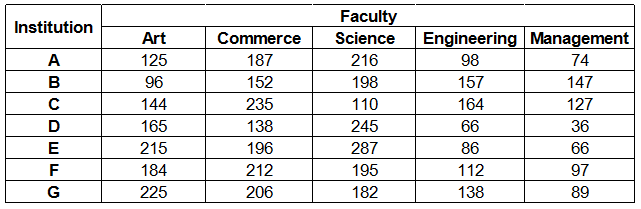### IBPS Clerk 2018 Question 16

Instructions

Study the following table carefully and answer the questions given below :
Number of students studying in different faculties in Seven institutionsQuestion 16

# What is the percentage of students studying Science to the total number of students studying in the institute G ?

Solution

Number of students studying Science in institute G = 182

Total number of students studying in the institute G

= 225 + 206 + 182 + 138 + 89 = 840

=> Required % = $$\frac{182}{840} \times 100$$

= $$\frac{13}{3} \times 5 = \frac{65}{3}$$

= $$21\frac{2}{3} \%$$